Tamilnadu State Board New Syllabus Samacheer Kalvi 12th Maths Guide Pdf Chapter 8 Differentials and Partial Derivatives Ex 8.4 Textbook Questions and Answers, Notes.

## Tamilnadu Samacheer Kalvi 12th Maths Solutions Chapter 8 Differentials and Partial Derivatives Ex 8.4

Question 1.
Find the partial dervatives of the following functions at indicated points.
(i) f(x, y) = 3x² – 2xy + y² + 5x + 2, (2, -5)
(ii) g(x, y) = 3x² + y² + 5x + 2, (2, -5)
(iii) h(x, y, z) = x sin (xy) + z² x, (2, π/4, 1)
(iv) G(x, y) = ex + 3y log (x² + y²), (-1, 1)
Solution:
(i) f(x, y) = 3x² – 2xy + y² + 5x + 2, (2, -5)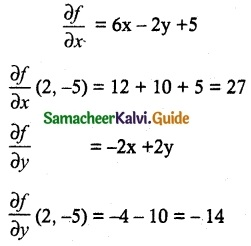(ii) g(x, y) = 3x² + y² + 5x + 2, (2, -5)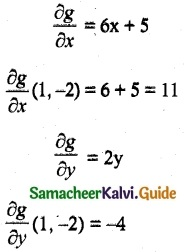(iii) h(x, y, z) = x sin (xy) + z² x, (2, π/4, 1)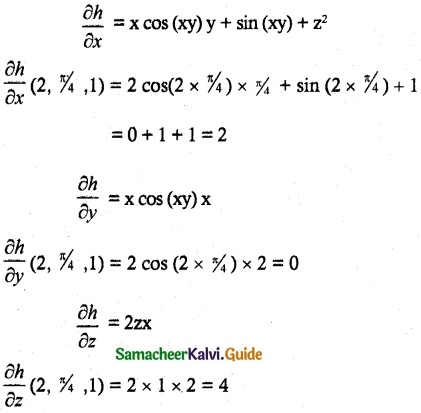(iv) G(x, y) = ex + 3y log (x² + y²), (-1, 1)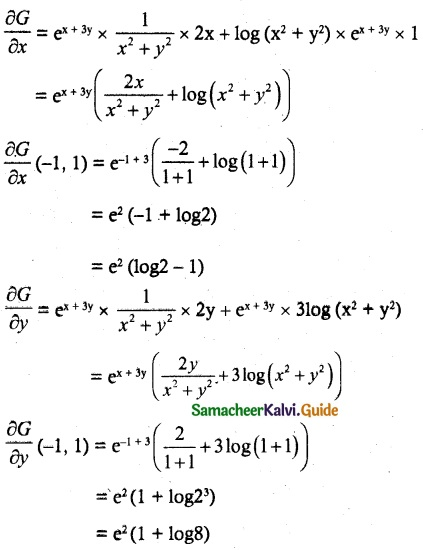Question 2.
For each of the following functions find the fx, and fy and show that fxy = fyx
(i) f(x, y) = $$\frac { 3x }{ y+sinx }$$
(ii) f(x, y) = tan-1(x/y)
(iii) f(x, y) = cos (x² – 3xy)
Solution:
(i) f(x, y) = $$\frac { 3x }{ y+sinx }$$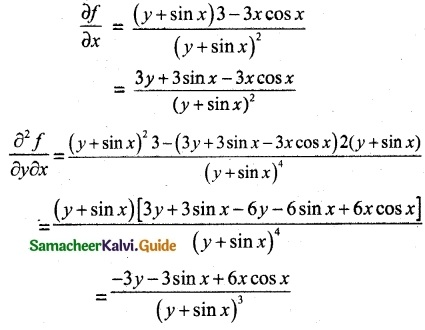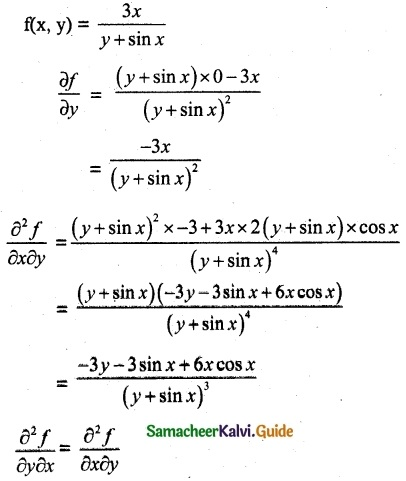(ii) f(x, y) = tan-1(x/y)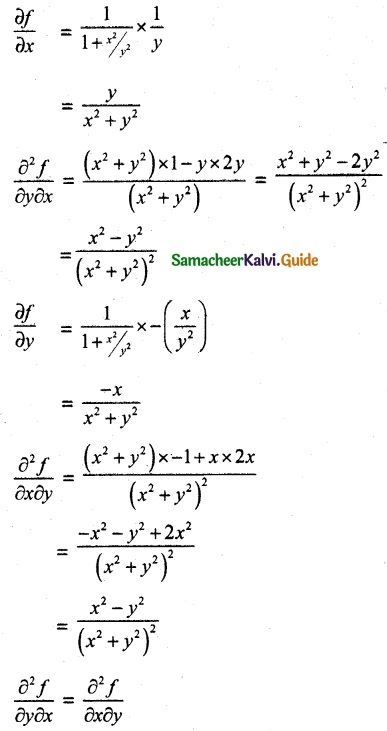(iii) f(x, y) = cos (x² – 3xy)
$$\frac{\partial f}{\partial x}$$ = -sin (x² – 3xy) × (2x – 3y)
$$\frac{\partial^2 f}{\partial y \partial x}$$ = – sin (x² – 3xy) x – 3 + (2x – 3y) × [-cos (x² – 3xy)] × – 3x
= 3 sin (x² – 3xy) + 3x (2x – 3y) cos (x² – 3xy)
$$\frac{\partial f}{\partial x}$$ = – sin (x² – 3xy) × -3x
= 3x sin (x² – 3xy)
$$\frac{\partial^2 f}{\partial x \partial y}$$ = 3sin (x² – 3xy) + 3x cos (x² – 3xy) (2x – 3y)
∴ $$\frac{\partial^2 f}{\partial y \partial x}$$ = $$\frac{\partial^2 f}{\partial x \partial y}$$Question 3.
If U (x, y, z) = $$\frac { x^2+y^2 }{ xy }$$ + 3z²y, find $$\frac{\partial U}{\partial x}$$, $$\frac{\partial U}{\partial y}$$ and $$\frac{\partial U}{\partial z}$$
Solution: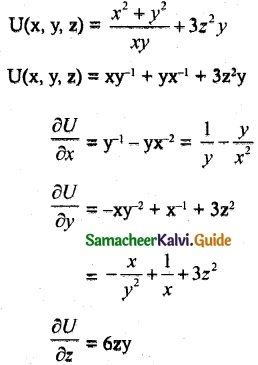Question 4.
If U(x, y, z) = log (x³ + y³ + z³) find
$$\frac{\partial U}{\partial x}$$ + $$\frac{\partial U}{\partial y}$$ + $$\frac{\partial U}{\partial z}$$
Solution: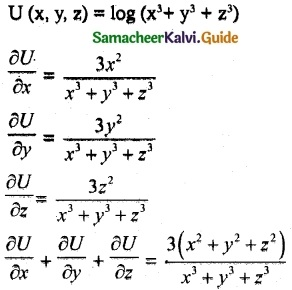Question 5.
For each of the following functions find the gxy, gxx, gyy and gyx
(i) g(x, y) = x ey + 3x²y
(ii) g(x, y) = log (5x + 3y)
(iii) g(x, y) = x² + 3xy – 7y + cos(5x)
Solution:
(i) g(x, y) = x ey + 3x²y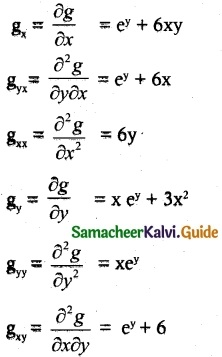(ii) g(x, y) = log (5x + 3y)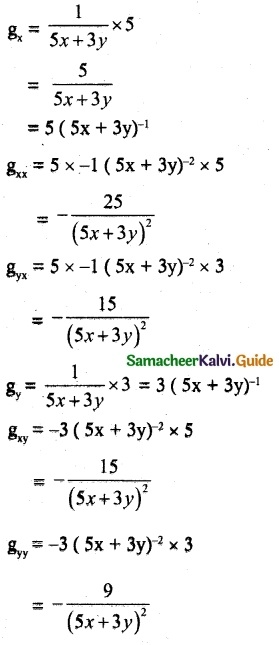(iii) g(x, y) = x² + 3xy – 7y + cos(5x)
gx = 2x+ 3y – sin 5x × 5
gx = 2x+ 3y – 5 sin 5x
gxx = 2 – 25 cos 5x
gyx = 3
gy = 3x – 7
gyy = 0
gxy = 3Question 6.
Let w(x, y, z) = $$\frac { 1 }{ \sqrt{x^2+y^2+z^2} }$$ = 1, (x, y, z) ≠ (0, 0, 0), show that $$\frac{\partial^ w}{\partial x^2}$$ + $$\frac{\partial^2 w}{\partial y^2}$$ + $$\frac{\partial^2 w}{\partial z^2}$$ = 0
Solution: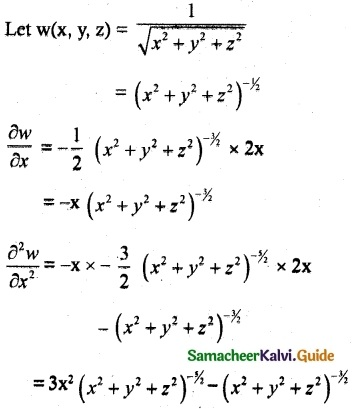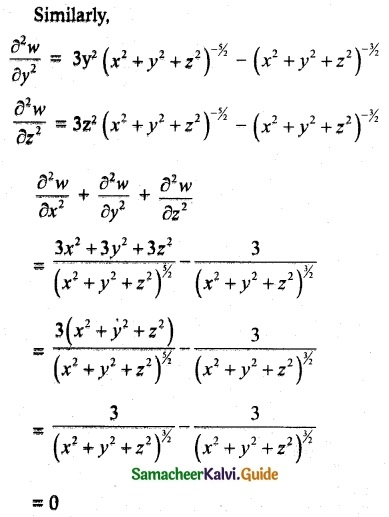Question 7.
If V (x, y) = ex ( x cosy – y siny), then Prove that $$\frac{\partial^2 V}{\partial x^2}$$ + $$\frac{\partial^2 V}{\partial y^2}$$ = 0
Solution: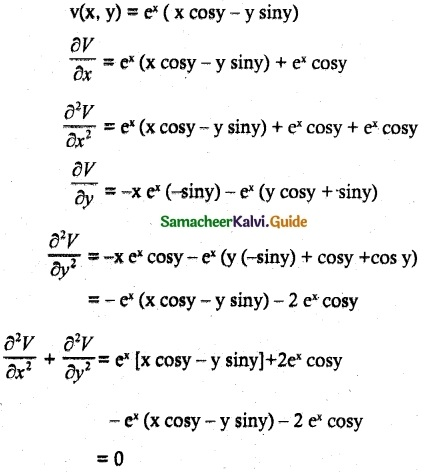Question 8.
If w (x, y) = xy + sin (xy), then Prove that $$\frac{\partial^2 w}{\partial y \partial x}$$ = $$\frac{\partial^2 w}{\partial x \partial y}$$
Solution:
Now, w (x, y) = xy + sin (xy)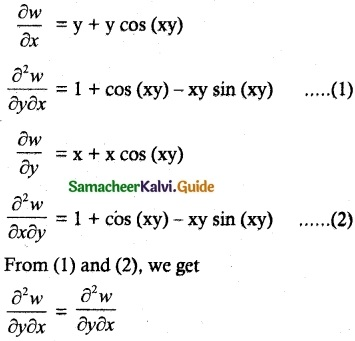Question 9.
If v(x, y, z) = x³ + y³ + z³ +3xyz, Show that $$\frac{\partial^2 v}{\partial y \partial z}$$ = $$\frac{\partial^2 v}{\partial z \partial y}$$
Solution:
v (x, y, z) = x³ + y³ + z³ + 3xyz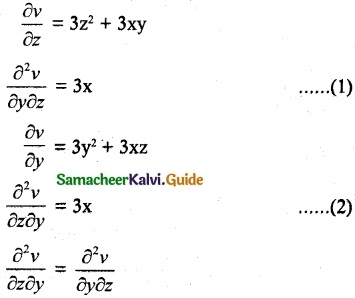Question 10.
A from produces two types of calculates each week, x number of type A and y number of type B. The weekly revenue and cost functions = (in rupees) are
R (x, y) = 80x + 90y + 0.04xy – 0.05x² – 0.05y² and C (x, y) = 8x + 6y + 2000 respectively.
(i) Find the Profit function P(x, y).
(ii) Find $$\frac{\partial P}{\partial x}$$ (1200, 1800) and $$\frac{\partial P}{\partial y}$$ (1200, 1800) and interpret these results.
Solution:
(i) Profit = Revenue – Cost
= (80x + 90y + 0.04 xy – 0.05 x2 – 0.05y2) – (8x + 6y + 2000)
= 80x + 90y + 0.04 xy – 0.05 x2 – 0.05y2 – 8x – 6y – 2000
P(x, y) = 72x + 84y + 0.04 xy – 0.05 x2 – 0.05y2 – 2000

(ii) $$\frac{\partial P}{\partial x}$$ = 72 + 0.04y – 0.1 x
$$\frac{\partial P}{\partial x}$$ (1200, 1800) = 72 + 0.04 × 1800 – 0.1 × 1200
= 72 + 72 – 120
= 144 – 120
= 24
$$\frac{\partial P}{\partial y}$$ = 84 + 0.04x – 0.1 y
$$\frac{\partial P}{\partial y}$$ (1200, 1800) = 84 + 0.04 × 1200 – 0.1 × 1800
= 84 + 48 – 180
= 132 – 180
= -48